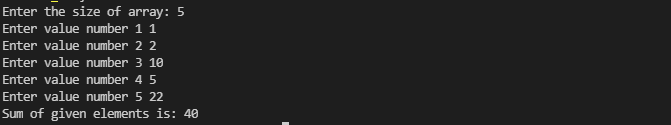Write a program that should ask the user to input the size of an array and then take that much number of values as input from the user and save them in the array. Finally, print the sum of all those values of the array.

Sample Program:

Enter the size of array: 5

Enter value number 1 1

Enter value number 2 2

Enter value number 3 10

Enter value number 4 5

Enter value number 5 22

The Sum of given elements is: 40

#include <iostream>
using namespace std;

int main()
{
int size;
int i = 0;
int sum = 0;
cout<<"Enter the size of array: ";
cin>>size;
int *arr = new int[size];
for (i = 0; i < size; i++)
{
cout<<"Enter value number "<<i+1<<" ";
cin>>arr[i];
sum += arr[i];
}
cout<<"The sum of given elements is: "<<sum;

return 0;
}cpp programming exercise with solutions